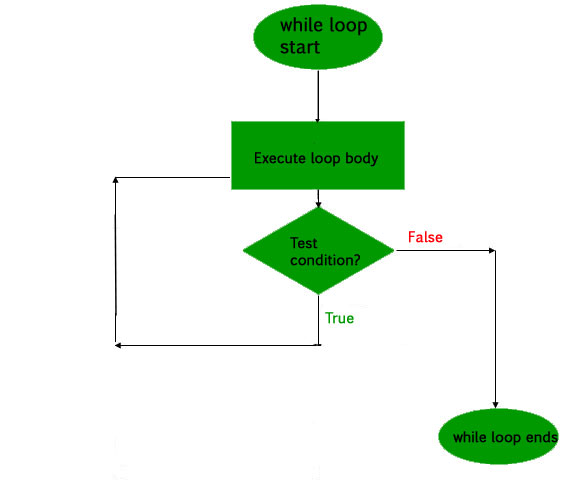# Kotlin do-while loop

Like Java, do-while loop is a control flow statement which executes a block of code at least once without checking the condition, and then repeatedly executes the block, or not, it totally depends upon a Boolean condition at the end of do-while block. It contrast with the while loop because while loop executes the block only when condition becomes true but do-while loop executes the code first and then the expression or test condition is evaluated.

do-while loop working –
First of the all the statements within the block is executed, and then the condition is evaluated. If the condition is true the block of code is executed again. The process of execution of code block repeated as long as the expression evaluates to true. If the expression becomes false, the loop terminates and transfers control to the statement next to do-while loop.

It is also known as post-test loop because it checks the condition after the block is executed.

Syntax of the do-while loop-

```do {
// code to run
{
while(condition)
```

Flowchart:Kotlin program to find the factorial of a number using do-while loop –

 `fun main(args: Array) { ` `    ``var number = ``6` `    ``var factorial = ``1` ` `  `    ``do` `{ ` `        ``factorial *= number ` `        ``number-- ` `    ``}``while``(number > ``0``) ` `    ``println(``"Factorial of 6 is \$factorial"``) ` `} `

Output:

`Factorial of 6 is 720`

Kotlin program to print table of 2 using do-while loop –

 `fun main(args: Array) { ` `    ``var num = ``2` `    ``var i = ``1` ` `  `    ``do` `{ ` `        ``println(``"2 * \$i = "``+ num * i) ` `        ``i++ ` `    ``}``while``(i < ``11``) ` `} `

Output:

```2 * 1 = 2
2 * 2 = 4
2 * 3 = 6
2 * 4 = 8
2 * 5 = 10
2 * 6 = 12
2 * 7 = 14
2 * 8 = 16
2 * 9 = 18
2 * 10 = 20
```
My Personal Notes arrow_drop_upCheck out this Author's contributed articles.

If you like GeeksforGeeks and would like to contribute, you can also write an article using contribute.geeksforgeeks.org or mail your article to contribute@geeksforgeeks.org. See your article appearing on the GeeksforGeeks main page and help other Geeks.

Please Improve this article if you find anything incorrect by clicking on the "Improve Article" button below.

Article Tags :

1

Please write to us at contribute@geeksforgeeks.org to report any issue with the above content.